## 15 Questions MCQ Test Quantitative Aptitude for GMAT | Test: Quadratic Equations- 3

Description
Attempt Test: Quadratic Equations- 3 | 15 questions in 30 minutes | Mock test for Quant preparation | Free important questions MCQ to study Quantitative Aptitude for GMAT for Quant Exam | Download free PDF with solutions
QUESTION: 1

### If x = 2 is a root of the quadratic equation 3x2 – px – 2 = 0, then the value of ‘p’ is

Solution: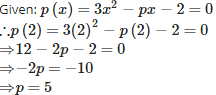QUESTION: 2

###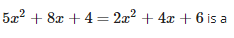Solution: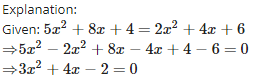Here, the degree is 2, therefore it is a quadratic equation.

QUESTION: 3

### If p = – 7 and q = 12 and x2+px+q=0, then the value of ‘x’ is

Solution: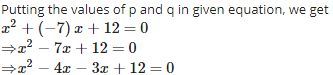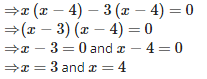QUESTION: 4

The roots of a quadratic equation x2−4px+4p2−q2=0 are

Solution: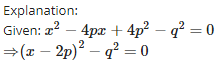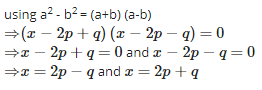QUESTION: 5

The two numbers whose sum is 27 and their product is 182 are

Solution:

Explanation:Let the one number be xx .As the sum  of numbers is 27 , then the other number will be (27−x)(27−x)                                                                                                                                    According to question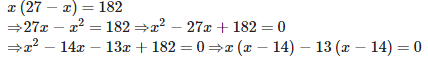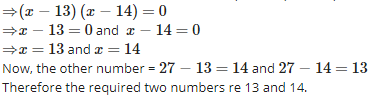QUESTION: 6

The common root of 2x2+x−6=0 and x2−3x−10=0 is

Solution: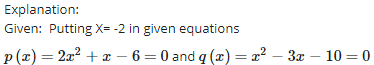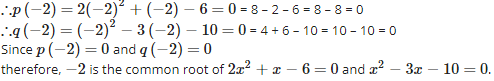QUESTION: 7

In a cricket match Kumble took three wickets less than twice the number of wickets taken by Srinath. The product of the number of wickets taken by these two is 20, then the number of wickets taken by Kumble is

Solution:

Explanation:

Let the number of wickets taken by Srinath be x then the number of wickets taken by Kumble will be 2x−3
According to question, x(2x−3)=20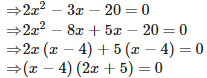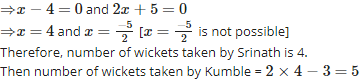QUESTION: 8

(x2 + 1)2 - x2 = 0 has

Solution: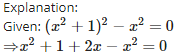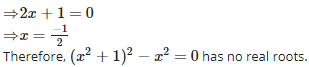QUESTION: 9

If the sum and product of the roots of the equation kx2 + 6x + 4k = 0 are equal, then k =

Solution: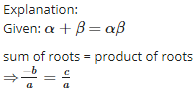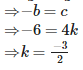QUESTION: 10

The product of two successive integral multiples of 5 is 1050. Then the numbers are

Solution:

Explanation:

Let one multiple of 5 be x then the next consecutive multiple of will be (x+5) According to question,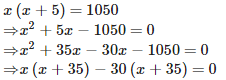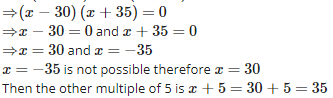Then the number are 30 and 35.

QUESTION: 11

The angry Arjun carried some arrows for fighting with Bheeshma. With half the arrows, he cut down the arrows thrown by Bheeshma on him and with six other arrows he killed the rath driver of Bheeshma. With one arrow each he knocked down respectively the rath, flag and bow of Bheeshma. Finally with one more than four times the square root of arrows he laid Bheeshma unconscious on an arrow bed. The total number of arrows that Arjun had is

Solution: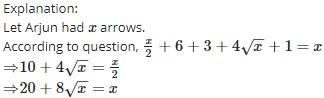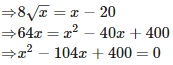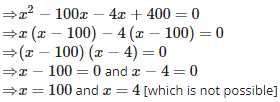QUESTION: 12

Which of the following has no real root?

Solution: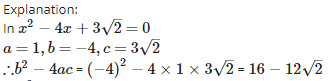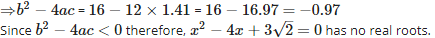QUESTION: 13

If 'sin α' and 'cos α' are the roots of thr equation ax2 + bx + c = 0, then b2 =

Solution: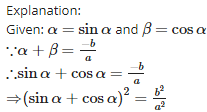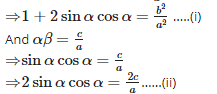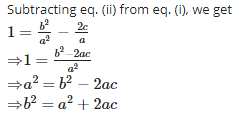QUESTION: 14

The constant that must be added and subtracted to solve the quadratic equation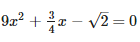by the method of completing the square is

Solution: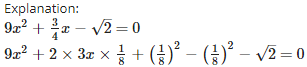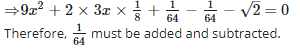QUESTION: 15

Which of the following has two distinct roots?

Solution: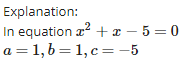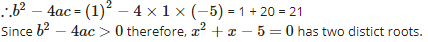Use Code STAYHOME200 and get INR 200 additional OFF Use Coupon Code